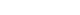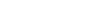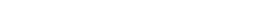xProbable primeEncyclopedia
In number theory
Number theory
Number theory is a branch of pure mathematics devoted primarily to the study of the integers. Number theorists study prime numbers as well...

, a probable prime (PRP) is an integer
Integer
The integers are formed by the natural numbers together with the negatives of the non-zero natural numbers .They are known as Positive and Negative Integers respectively...

that satisfies a specific condition also satisfied by all prime numbers. Different types of probable primes have different specific conditions. While there may be probable primes that are composite (called pseudoprime
Pseudoprime
In number theory, the Fermat pseudoprimes make up the most important class of pseudoprimes that come from Fermat's little theorem.- Definition :...

s), the condition is generally chosen in order to make such exceptions rare.

Fermat's test for compositeness, which is based on Fermat's little theorem
Fermat's little theorem
Fermat's little theorem states that if p is a prime number, then for any integer a, a p − a will be evenly divisible by p...

, works as follows: given an integer n, choose some integer a coprime
Coprime
In number theory, a branch of mathematics, two integers a and b are said to be coprime or relatively prime if the only positive integer that evenly divides both of them is 1. This is the same thing as their greatest common divisor being 1...

to n and calculate an − 1 modulo
Modular arithmetic
In mathematics, modular arithmetic is a system of arithmetic for integers, where numbers "wrap around" after they reach a certain value—the modulus....

n. If the result is different from 1, n is composite. If it is 1, n may or may not be prime; n is then called a (weak) probable prime to base a.

## Properties

Probable primality is a basis for efficient primality testing algorithm
Algorithm
In mathematics and computer science, an algorithm is an effective method expressed as a finite list of well-defined instructions for calculating a function. Algorithms are used for calculation, data processing, and automated reasoning...

s, which find application in cryptography
Cryptography
Cryptography is the practice and study of techniques for secure communication in the presence of third parties...

. These algorithms are usually probabilistic
Randomized algorithm
A randomized algorithm is an algorithm which employs a degree of randomness as part of its logic. The algorithm typically uses uniformly random bits as an auxiliary input to guide its behavior, in the hope of achieving good performance in the "average case" over all possible choices of random bits...

in nature. The idea is that while there are composite probable primes to base a for any fixed a, we may hope there exists some fixed P<1 such that for any given composite n, if we choose a randomly the probability that n is pseudoprime to base a is at most P. If we repeat this test k times, choosing a new a each time, the probability of n being pseudoprime to all the as tested is hence at most Pk, and as this decreases exponentially, only moderate k is required to make this probability negligibly small (compared to, for example, the probability of computer hardware error).

This is unfortunately false for weak probable primes, because there exist Carmichael numbers; but it is true for more refined notions of probable primality, such as strong probable primes (P = 1/4, Miller–Rabin algorithm), or
Euler probable primes (P = 1/2, Solovay–Strassen algorithm).

Even when a deterministic primality proof is required, a useful first step is to test for probable primality. This can quickly eliminate (with certainty) most composites.

A PRP test is sometimes combined with a table of small pseudoprimes to quickly establish the primality of a given number smaller than some threshold.

## Variations

An Euler probable prime to base a is an integer that is indicated prime by the somewhat stronger theorem that for any prime p, a(p − 1)/2 equalsmodulo p, whereis the Legendre symbol
Legendre symbol
In number theory, the Legendre symbol is a multiplicative function with values 1, −1, 0 that is a quadratic character modulo a prime number p: its value on a quadratic residue mod p is 1 and on a quadratic non-residue is −1....

. An Euler probable prime which is composite is called an Euler–Jacobi pseudoprime to base a.

This test may be improved by using the fact that the only square roots of 1 modulo a prime are 1 and −1. Write n = d · 2s + 1, where d is odd. The number n is a strong probable prime (SPRP) to base a if one of the following conditions holds:A composite strong probable prime to base a is called a strong pseudoprime
Strong pseudoprime
In number theory, a strong pseudoprime is a composite number that passes a primality test. All primes pass this test, but a small fraction of composites pass as well, making them "false primes"...

to base a. Every strong probable prime to base a is also an Euler probable prime to the same base, but not vice versa.

• Euler–Jacobi pseudoprime
• Carmichael number
• Miller–Rabin primality test
• Provable prime
Provable prime
In number theory, a provable prime is an integer that is calculated to be prime using a primality-proving algorithm. Contrast with probable prime, which is likely to be prime, based on the output of a probabilistic algorithm. There are algorithms that prove the primality of an integer. AKS...